# turbulence

(redirected from Turbulent fluid)
Also found in: Dictionary, Thesaurus, Medical.

## turbulence,

state of violent or agitated behavior in a fluid. Turbulent behavior is characteristic of systems of large numbers of particles, and its unpredictability and randomness has long thwarted attempts to fully understand it, even with such powerful tools as statistical mechanicsstatistical mechanics,
quantitative study of systems consisting of a large number of interacting elements, such as the atoms or molecules of a solid, liquid, or gas, or the individual quanta of light (see photon) making up electromagnetic radiation.
. Although much is still unknown about turbulence, recent developments in nonlinear dynamicsnonlinear dynamics,
study of systems governed by equations in which a small change in one variable can induce a large systematic change; the discipline is more popularly known as chaos (see chaos theory).
have led to an understanding of the onset of turbulence, and the advent of the supercomputer has enabled better models of turbulent states to be developed. Until the early 1970s, it was held that laminar, or smooth, flow made a gradual transition to turbulent flow by the addition of instabilities, one at a time, until the flow became unpredictable. Experimental work, however, has shown that the onset of turbulence occurs abruptly, and in fact is characterized by the so-called strange attractors of nonlinear dynamics. Increased understanding of turbulent flow through supercomputer models is leading to advances in such diverse areas as the design of better airplane wings and artificial heart valves.
The following article is from The Great Soviet Encyclopedia (1979). It might be outdated or ideologically biased.

## Turbulence

a phenomenon that is observed in many flows of liquids and gases and consists in the formation of numerous eddies of different sizes in these flows. As a result of eddy formation, hydrodynamic and thermodynamic characteristics of the flows, such as velocity, temperature, pressure, and density, undergo random fluctuations and therefore vary irregularly from point to point and in time. Such fluctuations distinguish turbulent flows from laminar flows. Most flows of liquids and gases in nature and in man-made systems are turbulent. Examples of such flows in nature include the motion of the air in the earth’s atmosphere, of the water in rivers and seas, and of the gas in the atmospheres of the sun and stars and in interstellar nebulas; examples in man-made systems include flows in pipes and channels, in jets, in boundary layers around bodies moving through a liquid or gas, and in the wakes behind such bodies.

As a result of the great strength of turbulent mixing, turbulent flows have an elevated capability of transporting momentum (and therefore exerting an increased force on bodies immersed in a flow), of transporting heat, and of accelerating the rate of chemical reactions, particularly combustion. Such flows can also, for example, carry suspended particles, scatter sound waves and electromagnetic waves, cause the phases and amplitudes of such waves to fluctuate, and—in the case of an electrically conducting fluid—generate a fluctuating magnetic field.

Turbulence arises as a result of some hydrodynamic instability of a laminar flow, which becomes unstable and undergoes a transition to a turbulent flow when the Reynolds number Re = Iv/v exceeds some critical value Recr, where l and v are the characteristic length and velocity, respectively, in the flow of interest and v is the kinematic viscosity. According to experimental data, in straight circular pipes with the highest possible degree of flow perturbation at the pipe inlet, Recr ≈ 2,300, where l is the pipe diameter and v is the average mean velocity over a cross section of the pipe. By reducing the degree of the initial flow perturbation, we can extend the laminar flow regime to substantially larger Recr, for example, up to Recr ≈ 50,000 in pipes. Similar results have been obtained for the onset of turbulence in a boundary layer.

The onset of turbulence in flows past bodies may be manifested not only as the occurrence of turbulence in a boundary layer but also as the formation of a turbulent wake behind the body as a result of boundary-layer separation from the body’s surface. The occurrence of turbulence in a boundary layer up to the point of separation results in a sharp decrease in the total resistance coefficient of the body. Turbulence may even arise far from solid walls when a velocity discontinuity surface becomes unstable or when the density distribution of fluid layers in a gravity field becomes unstable, that is, during the onset of convection. A velocity discontinuity surface is, for example, a surface formed during boundary-layer separation, the edge of a submerged jet, or a density discontinuity surface. Lord Rayleigh established that the condition for the onset of convection in a fluid layer of thickness h between two planes with a temperature difference or has the form Ragβh3δT/vX, where g is the acceleration of gravity, B is the thermal expansion coefficient of the fluid, and x is the fluid’s thermal diffusivity. The critical Rayleigh number Racr has a value of about 1,100–1,700.

Because of the extraordinary irregularity of the hydrodynamic fields of turbulent flows, a statistical description of turbulence is used in which the hydrodynamic fields are treated as stochastic functions of space-time points and the probability distributions are studied for the values of these functions in finite ensembles of such points. The following simple characteristics of the distributions are of the greatest practical interest: the mean values and the second moments of the hydrodynamic fields, including the variances of the velocity components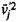, whererepresents the velocity pulsations (the bar above the vj indicates averaging); the components of the turbulent momentum flux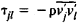(the Reynolds stresses); and the components of the turbulent heat flux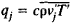(where ρ is the density, c is the specific heat, and T is the temperature). The statistical moments of the hydrodynamic fields of a turbulent flow should satisfy certain equations, which follow from the equations of hydrodynamics; the simplest such equations, called the Reynolds equations, are obtained by averaging the equations of hydrodynamics. Since, however, an exact solution has not yet been found for these equations, various approximate methods are used.

The large-scale components of turbulence, whose scales are comparable to those of the flow as a whole, make the principal contribution to the transport of momentum and heat through a turbulent medium. The description of these components is therefore the basis for calculations of resistance and heat exchange during the flow of a liquid or gas around bodies. A number of semiempirical turbulence theories have been devised for this purpose. In these theories, an analogy between turbulent and molecular transport is used; the concepts of the mixing length, turbulent intensity, eddy viscosity, and thermal conductivity are introduced; and hypotheses are adopted concerning the existence of linear relations between the Reynolds stresses and the mean strain rates, the turbulent heat flux, and the mean temperature gradient. An example of such a theory is the Boussinesq approximation τ = Adv/dy, which is used for two-dimensional parallel averaged motion. This approximation has a turbulent mixing coefficient (eddy viscosity) A, which, in contrast to the molecular viscosity, is not a physical constant of the fluid but depends on the nature of the averaged motion. On the basis of Prandtl’s semiempirical theory it may be assumed that A = ρl2|dv̄/dy|, where the mixing length l is the turbulence analogue of the molecular mean free path.

Similarity hypotheses (seeSIMILARITY THEORY) play a major role in the semiempirical theories. In particular, such hypotheses form the basis for Kármán’s semiempirical theory, according to which the mixing length in a two-dimensional parallel flow has the form l = – κv′/v” where v = v(y) is the flow velocity and K is a constant. A. N. Kolmogorov proposed the use in semiempirical theories of a similarity hypothesis according to which the characteristics of turbulence are expressed in terms of the turbulent intensity b and scale t (for example, the rate of energy dissipation є ~ b3/l). One of the most important achievements of semiempirical turbulence theory is the establishment of a universal (with respect to the Reynolds number, at large Re) logarithmic law for the velocity profile in pipes, channels, and boundary layers

v(y)/v* = A log (y/y0) + B

which is valid at distances v from the wall that are not too small; here,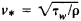w is the shearing stress at the wall), A and B are constants, and y0 = v/v* in the case of a smooth wall and is proportional to the geometric height of the roughness elements in the case of a rough wall.

The small-scale components of turbulence, that is, those whose scale is small in comparison with the scale of the flow as a whole, make a substantial contribution to, for example, the accelerations of the fluid particles, the capability (which is determined by the accelerations) of the turbulent flow for carrying suspended particles, the relative scattering of particles, the breakup of droplets in the flow, the mixing of turbulent fluids, the generation of a magnetic field in an electrically conducting fluid, the spectrum of electron-density inhomogeneities in the ionosphere, fluctuations of the parameters of electromagnetic waves, and air turbulence in aircraft flight.

The description of the small-scale components of turbulence is based on Kolmogorov’s hypotheses, which are grounded in the concept of a cascade process of energy transfer from large-scale to smaller-scale components of turbulence. Because of the randomness and multicascade nature of this process at very large Re, the system of small-scale components is spatially homogeneous, isotropic, and quasi-steady-state and is determined by the presence of a mean energy flux ε̄ from the large-scale components and an equal mean energy dissipation in the region of the smallest scales. According to Kolmogorov’s first hypothesis, the statistical characteristics of the small-scale components are determined by only two parameters: e and v; in particular, the minimum scale of turbulent inhomogeneities is λ ~ (v3/ε̄)¼ (in the atmosphere λ ~ 10–1 cm). According to his second hypothesis, at very large Re in the small-scale region there exists a range, called the inertial range, of scales that are large in comparison with λ. In this range the parameter v is insignificant, so that the characteristics of turbulence in this range are determined solely by the single parameter ε̄.

The theory of the similarity of the small-scale components of turbulence has been used to describe the local structure of temperature fields, pressure fields, acceleration fields, and the fields of passive impurities. The conclusions of the theory have been confirmed by measurements of the characteristics of various turbulent flows. In 1962, Kolmogorov and A. M. Obukhov proposed that the theory be improved by taking into account the fluctuations of the energy dissipation field. The statistical properties of these fluctuations are not universal; they may differ in different types of flows and, in particular, may depend on Re.

### REFERENCES

Monin, A. S., and A. M. Iaglom. Statisticheskaia gidromekhanika, part 1. Moscow, 1965. Part 2: Moscow, 1967.
Hinze, J. O. Turbulentnost. Moscow, 1963. (Translated from English.)
Townsend, A. A. Struktura turbulentnogo potoko s poperechnym sdvigom. Moscow, 1959. (Translated from English.)
Batchelor, G. K. Teoriia odnorodnoi turbulentnosti. Moscow, 1955. (Translated from English.)
Landau, L. D., and E. M. Lifshits. Mekhanika sploshnykh sred, 2nd ed. (Teoretich. fizika.) Moscow, 1954.
Lin Chia-ch’iao. Teoriia gidrodinamicheskoi ustoichivosti. Moscow, 1958. (Translated from English.)
Loitsianskii, L. G. Mekhanika zhidkosti i gaza, 3rd ed. Moscow, 1970.
Schlichting, H. Vozniknovenie turbulentnosti. Moscow, 1962. (Translated from German.)
Gidrodinamicheskaia neustoichivost’: Sb. statei. Moscow, 1964. (Translated from English.)
Tatarskii, V. I. Rasprostranenie voln v turbulentnoi atmosfere. Moscow, 1967.

A. S. MONIN

## turbulence

[′tər·byə·ləns]
(fluid mechanics)
McGraw-Hill Dictionary of Scientific & Technical Terms, 6E, Copyright © 2003 by The McGraw-Hill Companies, Inc.

## turbulence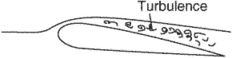i. The short period and small-scale oscillations in fluid flow.
ii. A state of fluid flow in which the instantaneous velocities exhibit irregular and apparently random fluctuations so that in practice only its statistical properties can be recognized.

## turbulence

(rarely), turbulency
1. Meteorol local instability in the atmosphere, oceans, or rivers
2. turbulent flow in a liquid or gas
Collins Discovery Encyclopedia, 1st edition © HarperCollins Publishers 2005
References in periodicals archive ?
If we imagine that turbulent fluid motion is in the form of coherent eddy structures (those where flows are rotational), this process can be envisioned as the breakup of larger eddies into a series of ever smaller structures.
The echo from a turbulent fluid surface being agitated may be significantly weaker than the echo from the agitator.
Intermittency is a feature of any odor plume that is generated in a turbulent fluid environment (Murlis, 1986, 1997; Murlis et al., 1992; Moore and Atema, 1991; Zimmer-Faust et al., 1995; Finelli et al., 1999).
RANS models replace all turbulent fluid dynamic effects with a turbulence model.
In the new work, Sirovich applied the pattern-analysis techniques that he had used previously to study turbulent fluid flow, face recognition, and the structure of the brain.
Free jets represent a very good benchmark for research in the physics of turbulent fluid flow.
125) addresses how much energy is given up in the viscous processes of a thin sheet of turbulent fluid. The technique used to stir the soap film grabbed my attention.
Using a soap film that mimics the atmosphere of Earth or other planets (SN: 8/22/98, p.118), physicists now have measured how much energy a thin sheet of turbulent fluid gives up to those types of losses.

Site: Follow: Share:
Open / Close